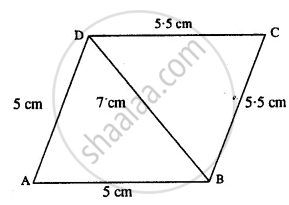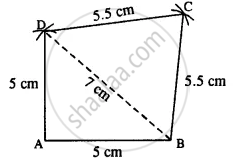# Construct a Quadrilateral Abcd; If: Ab = Ad = 5cm, Bd = 7 Cm and Bc = Dc = 5.5 Cm - Mathematics

Sum

AB = AD = 5cm, BD = 7 cm and BC = DC = 5.5 cm

#### Solution

The rough figure is as follow :Actual construction is as follows (using the above rough fig.)Steps :

1. Draw AB = 5 cm.
2. From A & B draw arcs of radii 5 cm and 7cm which intersect at D.
3. From B & D draw arcs of radii 5.5 cm each which intersect at C.
4. Join AD, BD, DC, and BC.
Thus ABCD is the required quadrilateral.
Is there an error in this question or solution?

#### APPEARS IN

Selina Concise Mathematics Class 8 ICSE
Chapter 18 Constructions
Exercise 18 (D) | Q 1.6 | Page 211